# Area of a convex quadrilateral. Example of creating a calculator.

This online calculator calculates area of a convex quadrilateral given diagonal values and value of angle between them. Article also describes the process of creating a calculator.

Visitors keep asking about a calculator which would compute the area of a convex quadrilateral given its sides. This is mathematically impossible. However, you can find a convex quadrilateral area, given quadrilateral diagonal values and the value of the angle between them.

Formula:

The calculator for the formula is below. After the calculator, I describe the process of creating a calculator using this site's engine.#### Area of a convex quadrilateral

Digits after the decimal point: 2

This site is an engine to create online calculators. All you need to create your own calculator is to be a registered user and understand basic Javascript syntax.

To create a calculator, you first should log in to the site. Then, in the "Personal section", you should choose the "Create" -> "Calculator" menu item.

You will get this form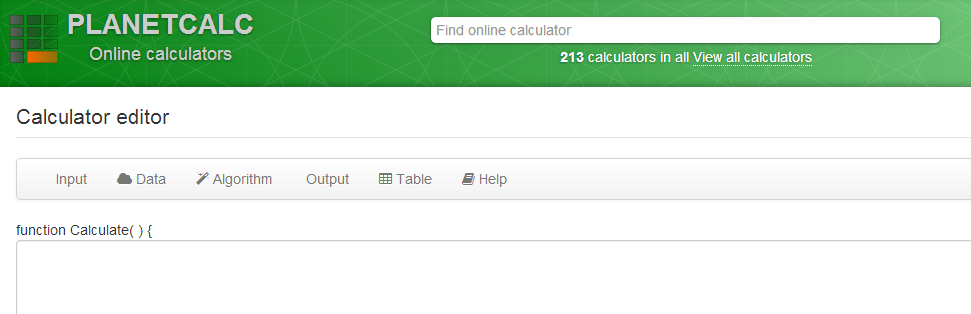The calculator, basically, is the thing, which receives user input and converts it to output values. So, for our calculator, we need three input parameters: two diagonals and an angle. We have to describe them for the engine.

Choose "Input" -> "Add new input parameter" in menu. You will get an input parameter editor, which should be filled as on the picture.Repeat for the second parameter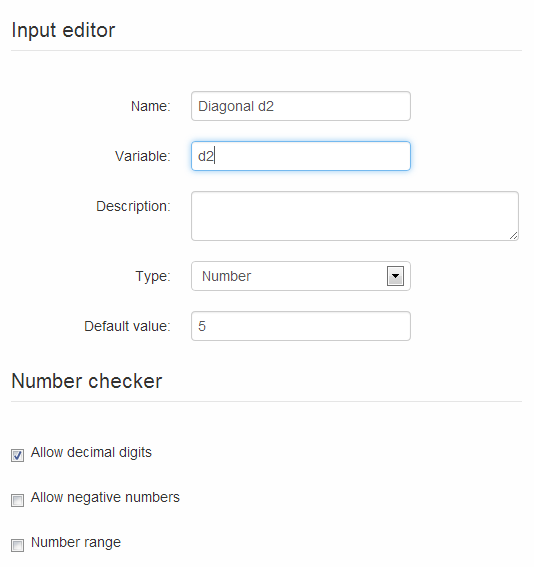For the third parameter, use range features and limit allowed values range from 0 to 180 degrees.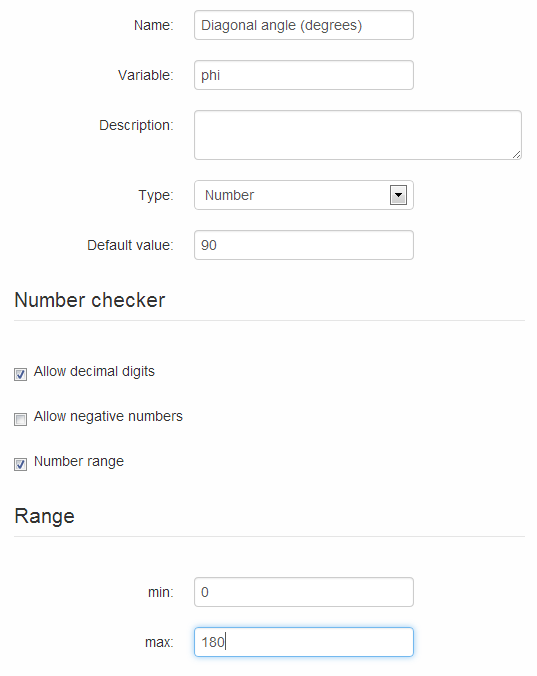Note that each time you change the parameters, the signature of function "Calculate" is changed to reflect those.

Now you have to describe the output parameter. Choose "Output" -> "New output parameter" and fill the form as below.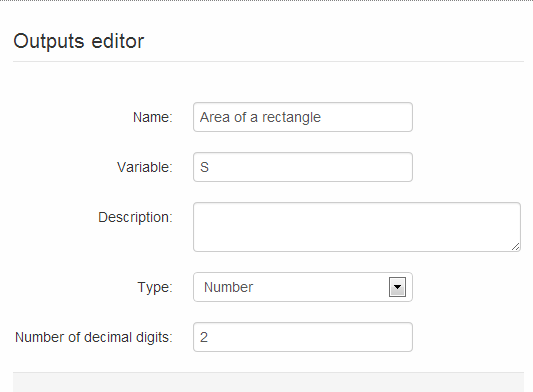Now we have described all ins and outs, so the only thing we need is to convert inputs to outputs inside the "Calculate" function. Here you will need Javascript.

Note that input parameters can be used directly. However, the output parameter is an object, and the only way to assign value to it is to use its special method, "SetValue".

Code which calculates area:The first line: note the conversion of degrees to radians and usage of Javascript built-in Math object.
The second line: note the usage of SetValue to assign calculation results.

Now you can press "Preview". This button allows you to preview and test the calculations and also saves the calculator source on site.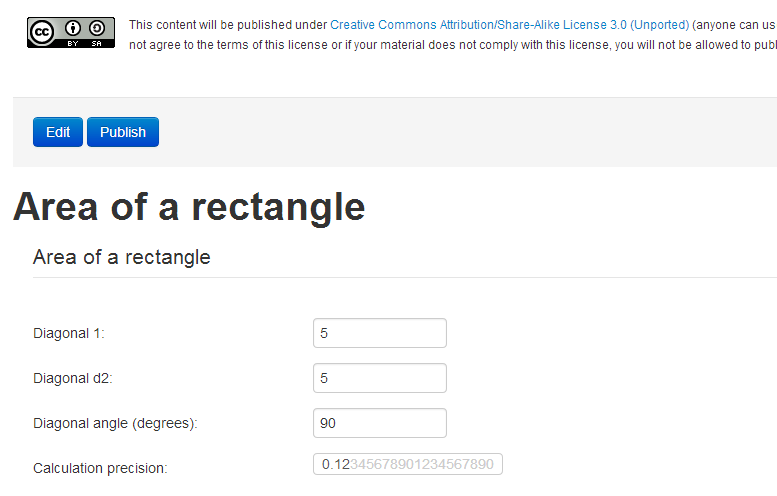Now you can publish the calculator using the button "Publish". After publishing, your calculator will get a constant URL, and the calculator will be available for everyone.

The final step is to create an article that describes the calculator. Article lets you educate visitors about your calculator, its purpose, and details of the calculation. To create an article, you should choose "Create" -> "Article" in the "Personal Section". Then you write some text and insert the calculator into your article, using the "Calculator" button on the toolbar.

URL copiado para a área de transferência